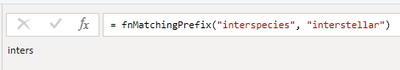cancel
Showing results for
Did you mean:Helper V

## How to get the Longest Common Prefix (LCP)?

Hi to all,

I have the need to get the longest common prefix between values in 2 columns. LCP is implemented in several languages in this rosettacode link, but is there a built-in function in M language? or how can I do it?

For example I found a function to combine 2 texts like this:

``````= Text.Combine({"interspecies","-","interstellar"})
interspecies-interstellar``````

But how would be a function to get this common prefix for the same 2 strings?

``````= SomeFuntion({"interspecies","interstellar"})
"inters"``````

2 ACCEPTED SOLUTIONSSolution Sage

Here you go.

``````(input1 as text, input2 as text)=>
let
Inputs = {input1, input2},
SplitAndZip = List.Zip(List.Transform(Inputs, each Text.ToList(_))),
CompareValues = List.Transform(SplitAndZip, each _{0} = _{1}),
FindFirstFalse = List.PositionOf(CompareValues, false),
KeepFirstCharacters = if input1=input2 then input1 else Text.Combine(List.FirstN(Text.ToList(Inputs{0}), FindFirstFalse), "")
in
KeepFirstCharacters``````

Pat

Microsoft EmployeeSolution Sage

Here you go.

``````(input1, input2)=>
let
Inputs = {input1, input2},
SplitAndZip = List.Zip(List.Transform(Inputs, each try Text.ToList(_) otherwise {})),
CompareValues = List.Transform(SplitAndZip, each _{0} = _{1}),
FindFirstFalse = List.PositionOf(CompareValues, false),
KeepFirstCharacters = if input1=input2 then input1 else Text.Combine(List.FirstN(Text.ToList(Inputs{0}), FindFirstFalse), "")
in
KeepFirstCharacters``````

Pat

Microsoft Employee
7 REPLIES 7Helper V

Thanks so much Pat, that fix the issue. RegardsHelper V

Thanks so much for your help. It almost working, only detected one issue when compares 2 strings that are equal. In this case the expected output should be the same string and currently is showing blank. Please see table below. For example some comparisons below and current and expected output.

 Input1 Input2 Current Output Expected Output 9000 9999 9 9 8549000 8549999 8549 8549 50 99 99999 99999 99999Solution Sage

Here you go.

``````(input1 as text, input2 as text)=>
let
Inputs = {input1, input2},
SplitAndZip = List.Zip(List.Transform(Inputs, each Text.ToList(_))),
CompareValues = List.Transform(SplitAndZip, each _{0} = _{1}),
FindFirstFalse = List.PositionOf(CompareValues, false),
KeepFirstCharacters = if input1=input2 then input1 else Text.Combine(List.FirstN(Text.ToList(Inputs{0}), FindFirstFalse), "")
in
KeepFirstCharacters``````

Pat

Microsoft EmployeeHelper V

Thanks so much. This time it fits all conditions. I was trying to add in wrong place the "if else" you added. I see in your solution, the "if else" should go inside one step, not outside.Solution Sage

Here's a function that shows one way to do that with two text value inputs. Just create a blank query, open the advanced editor and replace the text with the M code below. You could then use the function in a custom column (for example) and provide the two text column names as inputs.``````(input1 as text, input2 as text)=>
let
Inputs = {input1, input2},
SplitAndZip = List.Zip(List.Transform(Inputs, each Text.ToList(_))),
CompareValues = List.Transform(SplitAndZip, each _{0} = _{1}),
FindFirstFalse = List.PositionOf(CompareValues, false),
KeepFirstCharacters = Text.Combine(List.FirstN(Text.ToList(Inputs{0}), FindFirstFalse), "")
in
KeepFirstCharacters``````

Pat

Microsoft EmployeeHelper V

@ppm1 I talk to much early. There is still an error when trying with actual data when input1 and/or input2 have "null" value.

I get this error.

``````Expression.Error: We cannot convert the value null to type Text.
Details:
Value=
Type=[Type]``````

The custom column I'm adding is like this

``#"Added Custom" = Table.AddColumn(#"Step123", "Custom Col", each Text.Combine({[Col1],[Col2],fnMatchingPrefix([Col3],[Col4])})),``

May you help me to fix this part in order that function ouputs empty values when Col3 and/or Col4 be null? ThanksSolution Sage

Here you go.

``````(input1, input2)=>
let
Inputs = {input1, input2},
SplitAndZip = List.Zip(List.Transform(Inputs, each try Text.ToList(_) otherwise {})),
CompareValues = List.Transform(SplitAndZip, each _{0} = _{1}),
FindFirstFalse = List.PositionOf(CompareValues, false),
KeepFirstCharacters = if input1=input2 then input1 else Text.Combine(List.FirstN(Text.ToList(Inputs{0}), FindFirstFalse), "")
in
KeepFirstCharacters``````

Pat

Microsoft Employee